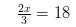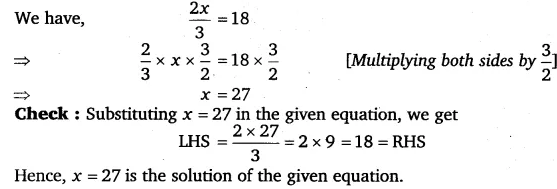# Solve the following equations: 2x/3 = 18

Solve the following equations: